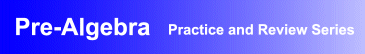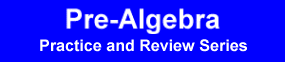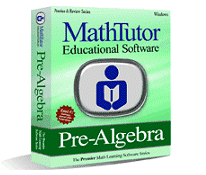Award-Winning

# Pre-Algebra Interactive Review and Practice SoftwareDESCRIPTION
PRE-ALGEBRA is a highly effective software package designed for review, drill and practice.
Designed by math curriculum specialists, PRE-ALGEBRA provides stimulating drill and practice exercises suitable for students at all levels of ability.
The program helps reinforce concepts through repetitive practice. Problems are randomized and based on students' skill levels.
PRE-ALGEBRA offers step by step explanations that help students understand how to apply the concepts they have learned to problem solving. Feedback and recordkeeping allow students to see the progress they have made.
Examples and problem solving exercises progress gradually from the simpler to the more challenging, allowing students to develop problem solving skills at a comfortable pace.
• Pre-algebra practice and review software developed by math educators
• Interactive, stimulating pre-algebra exercises, explanations and animations that reinforce student understanding of pre-algebra concepts
• Proven record of success helping students achieve mastery of pre-algebra problem solvingTOPICS COVERED
Integers
• The Number Line
• Multiplication Table
• Addition and Subtr. of Pos., Neg. Integers
• Multiplication of Positive Integers
• Word Problems: Addition, Subtraction
• Word Problems: Multiplication, Division
Fractions
• Least Common Multiple, Greatest Common Divisor
• Equivalent Fractions
• Addition and Subtraction of Fractions
• Addition and Subtraction of Mixed Numbers
• Mult. and Division of Fractions
Decimals
• Addition and Subtraction of Decimals
• Mult. and Division of Decimals
• Equivalent Expressions
• Scientific Notation
Variables
• Words and Symbols
• Words and Equations
• Word Problems: Books
• Statements and Equations
Expressions and Equations
• Substitution
• Satisfying Equations I
• Satisfying Equations II
• Correct Solutions
• Incorrect Equations
• Incorrect Solutions
Algebraic Rules
• Linear Terms I
• Linear Terms II
• Several Variables
• Products
• Simple Powers
• Inequalities
Problem Solving
• Necessary Information